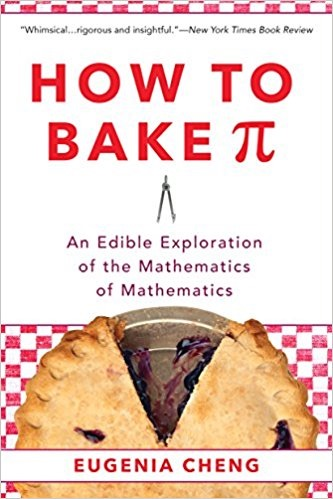## How To Bake ∏: An Edible Exploration of the Mathematics of Mathematics by Eugenia ChengHow To Bake ∏: An Edible Exploration of the Mathematics of Mathematics by Eugenia Cheng uses classic baking recipes to illuminate many math concepts. It helps take math into the real world as it helps to make some math seem easier to its readers. At the very least, it should find its way into the hands of every math teacher and serious math students.

### Part I: Math – 1. What Is Math?

• Like all chapters this one starts with a recipe since like recipes math has both ingredients and methods. In math, however, the method being used is probably more important than the ingredients. Once you find a technique in math you can always find more things to study with it. The two key concepts here are abstraction and generalization. Math is abstract in that it studies ideas of things, rather than real things. The invention of numbers to represent things was perhaps the first-ever process of abstraction.

### 2. Abstraction

• To do math you have to step away from reality. This is where math finds its strength. The idea that two things cost twice as much as one thing applies to all things, for example. Here we have a process that generalizes. Maps are abstractions of reality. The key for map makers is to find the most appropriate level of abstraction for the given moment. There are a number of math problems here that show how procedures work regardless of the specific numbers involved.

### 3. Principals

• When making any recipe, it helps to understand the principals of the ingredients and finished products you are working on. Understanding the principles allows you to take shortcuts and substitute ingredients without ruining everything. Recipe books, however, rarely explain such principles. Understanding is power in cooking and in math. At the heart of math is the desire to understand things rather than just knowing them. The chapter goes on to spell out the principals for the natural numbers we use for counting.

### 4. Process

• Flour, butter, water, and salt can make a delicious puff pastry, but only if you follow a specific method. Math requires the proper method also and further requires that almost nothing is basic or given. The rules of logic must be followed. Eugenia shows how you can make two mistakes in math and still get the correct answer. At the heart of math is the need to understand things rather than just knowing them. In other words, the means justifies the end.
DrDougGreen.com     If you like the summary, buy the book
Pages: 1 2 3